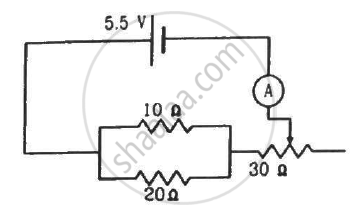Department of Pre-University Education, KarnatakaPUC Karnataka Science Class 12
Advertisement Remove all ads

# The Resistance of the Rheostat Shown in the Figure is 30 ω. Neglecting the Meter Resistance, Find the Minimum and Maximum Currents Through the Ammeter as the Rheostat is Varied. - Physics

Sum

The resistance of the rheostat shown in the figure is 30 Ω. Neglecting the meter resistance, find the minimum and maximum currents through the ammeter as the rheostat is varied.Advertisement Remove all ads

#### Solution

Current will be minimum when the rheostat will have maximum resistance, i.e 30 Ω.

In this case, total resistance of the circuit = $\frac{20 \times 10}{30} + 30 = 36 . 67 \Omega$

The minimum current through the circuit,

$i_\min = \frac{5 . 5}{36 . 67} = 0 . 15 A$

Current will be maximum when the rheostat will have minimum resistance, i.e. 0 Ω.

In this case, total resistance of the circuit = $\frac{20 \times 10}{30} = 6 . 67 \Omega$

The maximum current through the circuit,

$i_\max = \frac{5 . 5}{6 . 67} = 0 . 825 A$

Is there an error in this question or solution?
Advertisement Remove all ads

#### APPEARS IN

HC Verma Class 11, Class 12 Concepts of Physics Vol. 2
Chapter 10 Electric Current in Conductors
Q 24 | Page 199
Advertisement Remove all ads

#### Video TutorialsVIEW ALL 

Advertisement Remove all ads
Share
Notifications

View all notifications

Forgot password?